In what quadrant is a -1165° angle?

x =  4

### Step-by-step explanation: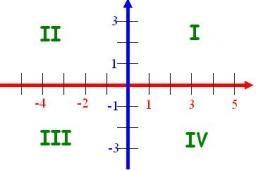Did you find an error or inaccuracy? Feel free to write us. Thank you!Tips to related online calculators
Do you want to round the number?

## Related math problems and questions: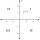Which point is located in Quadrant IV? A coordinate plane. A(-8, 6) B(-8, -6) C(8, -6) D(8, 6)Calculate the radius of the quadrant, which area is equal to area of circle with radius r = 15 cm.
• Angle at the apex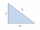In an isosceles triangle, the angle at the apex is 30° greater than the angle at the base. How big are the internal angles?
• Shooter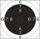The shooter fired at a target from a distance 11 m. The individual concentric circle of targets has radius increments of 1 cm (25 points) by 1 point. The shot was shifted by 8' (angle degree minutes). How many points should win his shot?
• No. of divisorsHow many different divisors have number 13 4 * 2 4?
• Isosceles triangle 8If the rate of the sides an isosceles triangle is 7:6:7, find the base angle correct to the nearest degree.
• Internal anglesFind the internal angles of the triangle ABC if the angle at the vertex C is twice the angle at the B and the angle at the vertex B is 4 degrees smaller than the angle at vertex A.
• LiftThe largest angle at which the lift rises is 16°31'. Give climb angle in permille.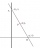On a graph, Point Y is located at (4, -2). Point Z is located 5 units to the left of Point Y. In which quadrant is Point Z located?
• n-gon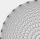Gabo draws n-gon, which angles are consecutive members of an arithmetic sequence. The smallest angle is 70° biggest 170°. How many sides have Gabo's n-gon?
• DivisibilityDetermine the smallest integer which divided 11 gives remainder 4 when divided 15 gives remainder 10 and when divided by 19 gives remainder 16.
• RiverFrom the observatory 11 m high and 24 m from the riverbank, river width appears in the visual angle φ = 13°. Calculate the width of the river.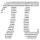Convert 270° to radians. Write result as multiple of number π.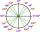What is greater angle? -0.7 radians or -0.7π radians?The size of the angles of the triangle are in ratio x: y = 7: 5 and the angle z is 42° lower than the angle y. Find size of the angles x, y, z.The triangle is ratio of the angles β:γ = 6:8. Angle α is 40° greater than β. What are the size of angles of the triangle?Mast has 13 m long shadow on a slope rising from the mast foot in the direction of the shadow angle at angle 13.3°. Determine the height of the mast, if the sun above the horizon is at angle 45°12'.